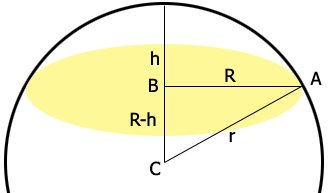SEARCH HOMEMath Central Quandaries & QueriesQuestion from Chithrabhanu: If a dom dia is 4.552 and the height is 1.21 what is the surface area?Hi,

In my diagram the radius of the base of the dome is $R,$ in your case 4.552/2 units, and H is its height, in your case h = 1.21 units. $B$ is the center of the base of the dome and $C$ is the center of the sphere that forms the dome. The radius of the sphere is $r$ units.The triangle $ABC$ is a right triangle and hence Pythagoras Theorem insures that

$R^2 + (R-h)^2 = r^{2}.$

Since you know $R$ and $h$ you can solve this equation for $r.$

Now use the expression in our response to an earlier question to calculate the surface area.

PennyMath Central is supported by the University of Regina and The Pacific Institute for the Mathematical Sciences.